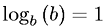Equations > Algebra > Logarithms > Logarithmic Identity Property

### Logarithmic Identity PropertyLatex Code:

MathML Code:

 ${\mathrm{log}}_{b}\left(b\right)=1$

MathType 5.0: